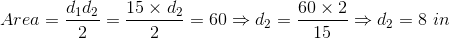# ISEE Upper Level Math : How to find the length of the diagonal of a kite

## Example Questions

### Example Question #1 : How To Find The Length Of The Diagonal Of A Kite

A kite has the area of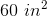. One of the diagonals of the kite has length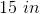. Give the length of the other diagonal of the kite.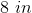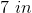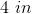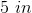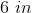Explanation:

The area of a kite is half the product of the diagonals, i.e.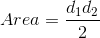,

where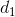and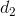are the lengths of the diagonals.